# ←blog

1. ## Learning about scalar numbers and multiprecision integers

### In pursuit of a JavaScript OpenPGP Implementation

#### Introduction

Recently I’ve been studying the OpenPGP specification in hopes of developing a Javascript implementation. I’m aware that someone has already done this, however I’m not a fan of using GPL-licensed code (I prefer more permissive MIT-style licensing). Furthermore, I have an academic curiosity towards learning and doing this myself, and thus I set out to learn all there is to know about PGP encryption.

Shortly into reading the spec, I realized that there is a lot of math and number theory involved in OpenPGP, and found that I’ve become rusty since dropping out of my math major (I left halfway through my undergrad cryptography course—d'oh!). Additionally, there is a lot of technical notation within the spec that is over and above anything I’ve encountered before. This isn’t the sort of stuff you can just search for on Google and quickly have the knowledge piped into your brain. There is much learning to be done, and not a lot of existing hand-holding online to help, and that’s why I’m writing this. Consider this an online version of my math notebook. If I can help you learn PGP in the process of helping myself, then great.

Disclaimer: I am not an authority on the subject of cryptography. I’m doing my best, but the information contained herein may be inaccurate, incomplete, or simply wrong. I encourage you to fact-check and work through the examples on your own. Please let me know if you find any errors in my posts.

#### Scalar and big-endian numbers

I assume you’ve read up to section 3 in the OpenPGP specification. Despite it being an ugly .txt file, it’s a surprisingly good read. You have a basic conceptual understanding of what PGP encryption is and how it works. Great. Well, section 3.1 is where it really starts getting technical, so let’s start off with a quote:

3.1 Scalar Numbers

Scalar numbers are unsigned and are always stored in big-endian format. Using n[k] to refer to the kth octet being interpreted, the value of a two-octet scalar is ((n << 8) + n). The value of a four-octet scalar is ((n << 24) + (n << 16) + (n << 8) + n).

Let’s pick this apart. An unsigned number means that it’s above zero, but what the hell is “big-endian format?” According to Wikipedia, a big-endian number has its most significant digits first. That’s easy—we use big-endian decimal numbers all the time in our daily lives. For example, if you find a sack containing \$1,024 in a dark alley, you know that 1 represents the thousands digit, 0 represents the hundreds digit, 2 represents the tens, and 4 represents the 1’s. Simple enough.

Now, we have to start thinking like a computer. An octet is a more specific way of saying a byte, or 8 binary bits. Be familiar with binary. It’s the most basic way a computer represents information and is a base-2 numeric notation (also in big-endian format) where each digit represents a power of two.

For example, decimal 187 can be represented by binary octet `10111011` as we see below:

##### 187 represented in binary: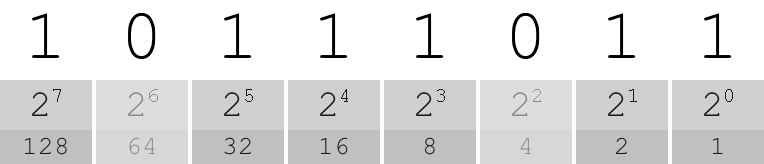Summing 128 + 32 + 16 + 8 + 2 + 1 = 187. Make sense? Getting back to the specification, we need to learn some notation. Specifically, how do we interpret:

the value of a two-octet scalar is ((n << 8) + n)

This is easiest to explain by way of example. Supposing you have a scalar number consisting of two octets `10111011` and `01000101` (69, lol). Respectively, these may be referred to by `n` and `n`. With `n << 8`, we perform the operation of shifting `n`’s binary representation over to the left by 8 binary places, thus multiplying `n`’s value by 2^8. This can best be visualized:

##### 10111011 << 8: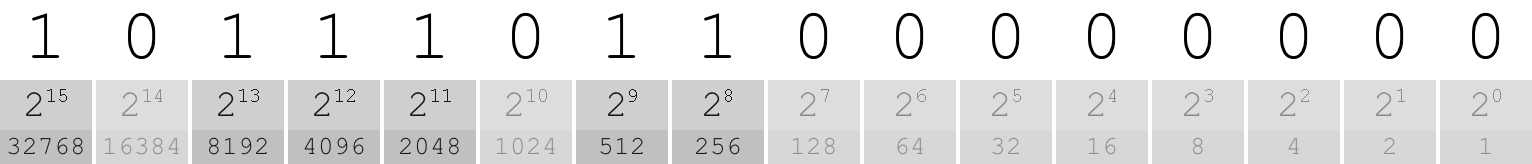Summing 32768 + 8192 + 4096 + 2048 + 512 + 256 = 47872 (which is 187 * 28). Now by adding in our value for `n`, we get 47872 + 69 = 47941. This can be represented in binary

##### (10111011 << 8) + 01000101: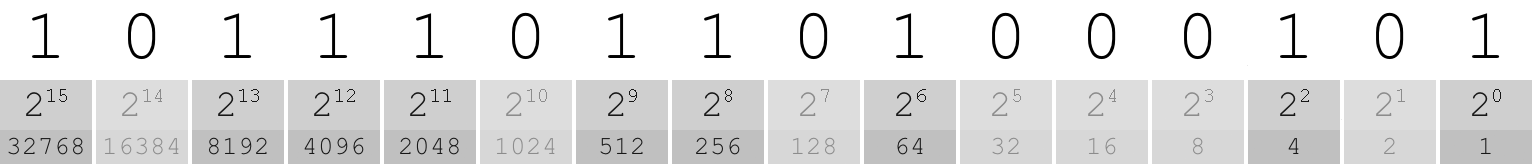In other words, we compute the value of a scalar number by taking the binary representations of each of its octets and smushing them together into one big long binary number. Whew.

#### Multiprecision Integers

3.2. Multiprecision Integers

Multiprecision integers (also called MPIs) are unsigned integers used to hold large integers such as the ones used in cryptographic calculations.

An MPI consists of two pieces: a two-octet scalar that is the length of the MPI in bits followed by a string of octets that contain the actual integer.

Section 3.2 gives a couple of examples, which I will explain, but first you’ll need a basic understanding of hexadecimal numbers. With binary, each digit (between 0 and 1) represents a power of two. With decimal, each digit (between 0 and 9) represents a power of 10. With hexadecimal, each digit (between 0 and …15?) represents a power of 16. Since we don’t have any numeric characters to represent numbers above 9, we start using letters of the alphabet! So we count 1, 2, 3, 4, 5, 6, 7, 8, 9, A, B, C, D, E, and F. lol

Here’s why we care about hexadecimal: it easily converts to binary for computer applications, since 16 is a power of 2, and it gives us a much more efficient way for notating octets than writing out their full binary representation. For example, we can write 249 in binary as:

##### 249 in binary: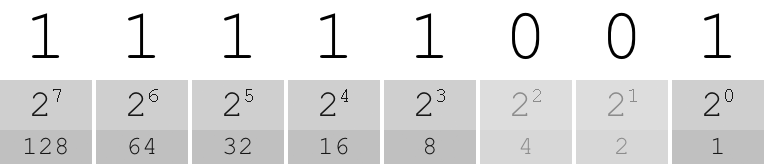But it's a hell of a lot more concise in hexadecimal:

##### 249 in hexadecimal: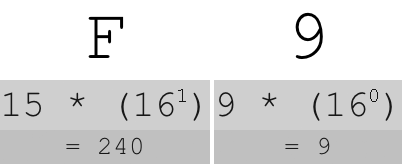Now, consider our first example using hexadecimal numbers in section 3.2:

The string of octets [00 01 01] forms an MPI with the value 1.

In this simple example, the first two octets `00 01` indicate the length of the multiprecision integer in bits, whereas the final `01` represents the actual integer. If we convert hexidecimal octets `00 01 01` to binary, we have `00000000 00000001 00000001`. So the first two octets tell us that the length of our MPI is 1 bit, and the final octet is the binary representation for the number 1 (which is indeed just 1 bit).

The string [00 09 01 FF] forms an MPI with the value of 511.

If we convert this to binary octets, we have `00000000 00001001 00000001 11111111`. The first two octets, again, form a scalar number indicating the length of the MPI in bits. Converting `00000000 00001001` to decimal, we see that our MPI is 9 bits long. The final two octets `00000001 11111111` represent the integer itself. We can smush these octets together into one binary scalar number `000000111111111` which has decimal representation 511. Notice that all significant digits occur in the first 9 bits (starting from the right), as indicated by our length scalar.

With the notation for multiprecision integers, we can represent extremely large integers (up to 65,536 bits), far beyond the typical 32-bit memory limit that a computer imposes on a regular integer value. This will become important later on in our cryptographic calculations, where we work with very large prime numbers.

Posted 2012-12-09 00:00:00 PST by henriquez.